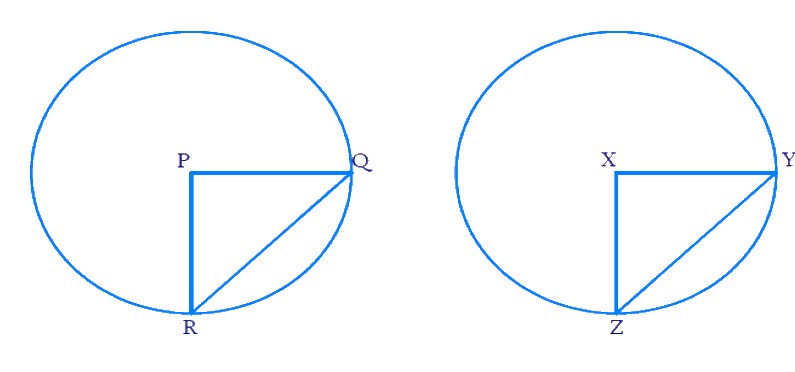# Ex.10.2 Q1 Circles Solution - NCERT Maths Class 9

Go back to  'Ex.10.2'

## Question

Recall that two circles are congruent if they have the same radii. Prove that equal chords of congruent circles subtend equal angles at their centers.

Video Solution
Circles
Ex 10.2 | Question 1

## Text Solution

What is known?

Two circles are congruent if they have same radii.

What is unknown?

Equal chords of congruent circles subtend equal angles at their centers.

Reasoning:

Using chords are equal and the fact that circles are congruent, we prove the statement using Side-Side-Side (SSS criteria) and Corresponding parts of congruent triangles (CPCT).

Steps:Let \begin{align} {QR} \end{align} and \begin{align} {YZ} \end{align} be the equal chords of $$2$$ congruent circles.

\begin{align} {QR = YZ} \end{align}

We need to prove that they subtend equal angles at centre. i.e. \begin{align}\angle{QPR} =\angle {YXZ}\end{align}

We know that the radii of both the circles are equal. So we get:

\begin{align} {PR = PQ = XZ = XY} \end{align}

Consider the $$2$$ triangles \begin{align} \Delta { PQR} \end{align} and \begin{align} \Delta { XYZ} \end{align}.

\begin{align} &{PQ = XY} \qquad \text{(Radii are equal)}\\&{PR = XZ} \qquad \text{(Radii are equal)}\\&{QR = YZ} \qquad \text{(Chords are equal)}\end{align}

By SSS criteria, \begin{align} \Delta\,{PQR}\end{align} is congruent to \begin{align} \Delta\;{XYZ}.\end{align}

So by CPCT (Corresponding parts of congruent triangles) we get \begin{align} \angle{QPR}=\angle{YXZ}.\end{align}

Hence proved that equal chords of congruent circles subtend equal angles at their centres.

Learn from the best math teachers and top your exams

• Live one on one classroom and doubt clearing
• Practice worksheets in and after class for conceptual clarity
• Personalized curriculum to keep up with school